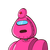# if p = 3 sec² and q = 3 tan² A – 1 , then find p – q??? please help meee​

if p = 3 sec² and q = 3 tan² A – 1 , then find p – q??? please help meee​

### 1 thought on “if p = 3 sec² and q = 3 tan² A – 1 , then find p – q??? please help meee​”

1.Step-by-step explanation:

p-q=3 sec²A -3 tan² A +1

= 3 – 3 sin²A +1

____ _____

cos²A. cos²A

= 3-3sin²A +cos²A

____________

cos²A

=3(1-sin²A) +cos²A

______________

cos²A

=3cos²A+cos²A

__________

cos²A

=4cos²A

_______

cos²A

=4